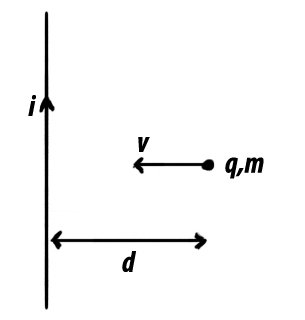# Come Closer!A Charged Particle of Mass $m$ and Charge $q$ is thrown with an initial velocity of $v$ towards an infinite wire carrying a current $i$ from an initial distance of $d$.

If the minimum separation (in meters) between the particle and the wire, during the course of time, is $\ell$, then Evaluate $\ell\times 10^{49}$ to the nearest Integer

Details and Assumptions:

• $\mu_o = 4\pi \times 10^{-7}\frac{\text{N}}{\text{A}^2}$
• $i = 5\text{ A}$
• $m = 0.08\text{ g}$
• $d = 4\text{ m}$
• $v = 1.386\frac{\text{m}}{\text{s}}$
• $q = 1\text{ C}$
×